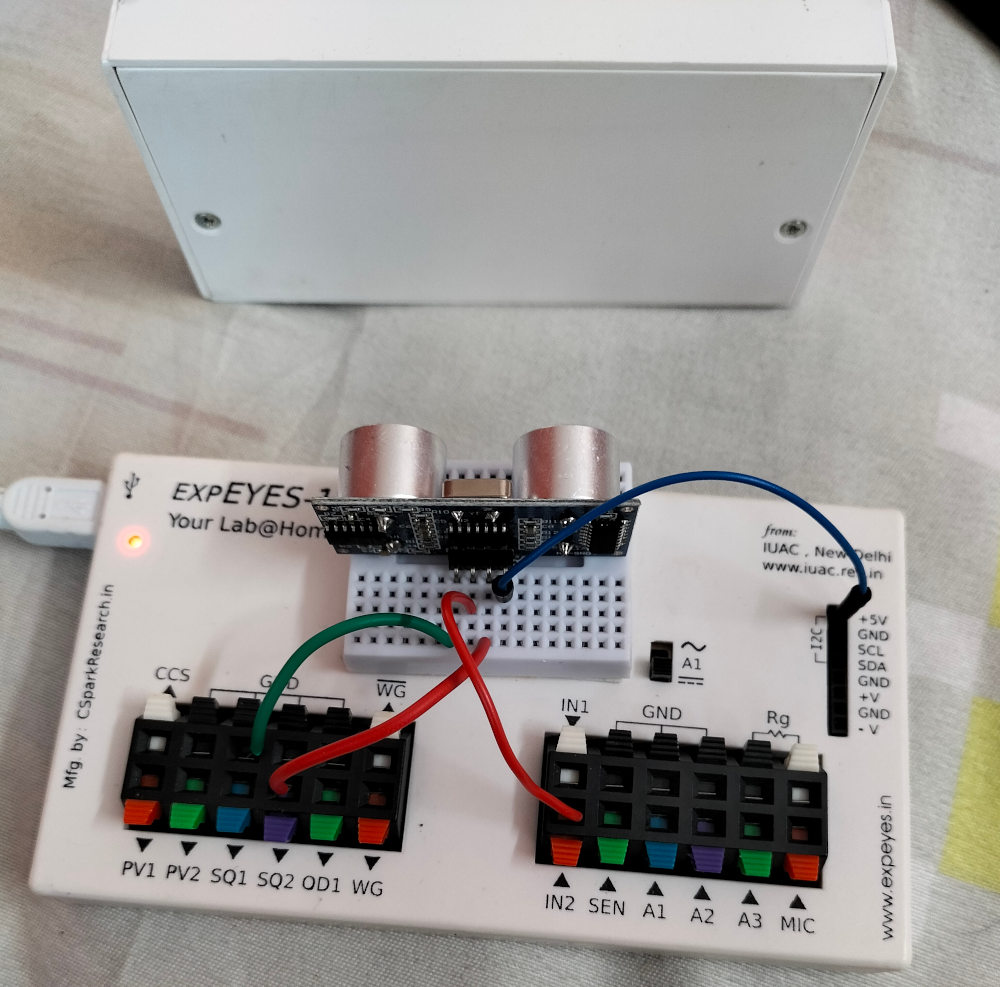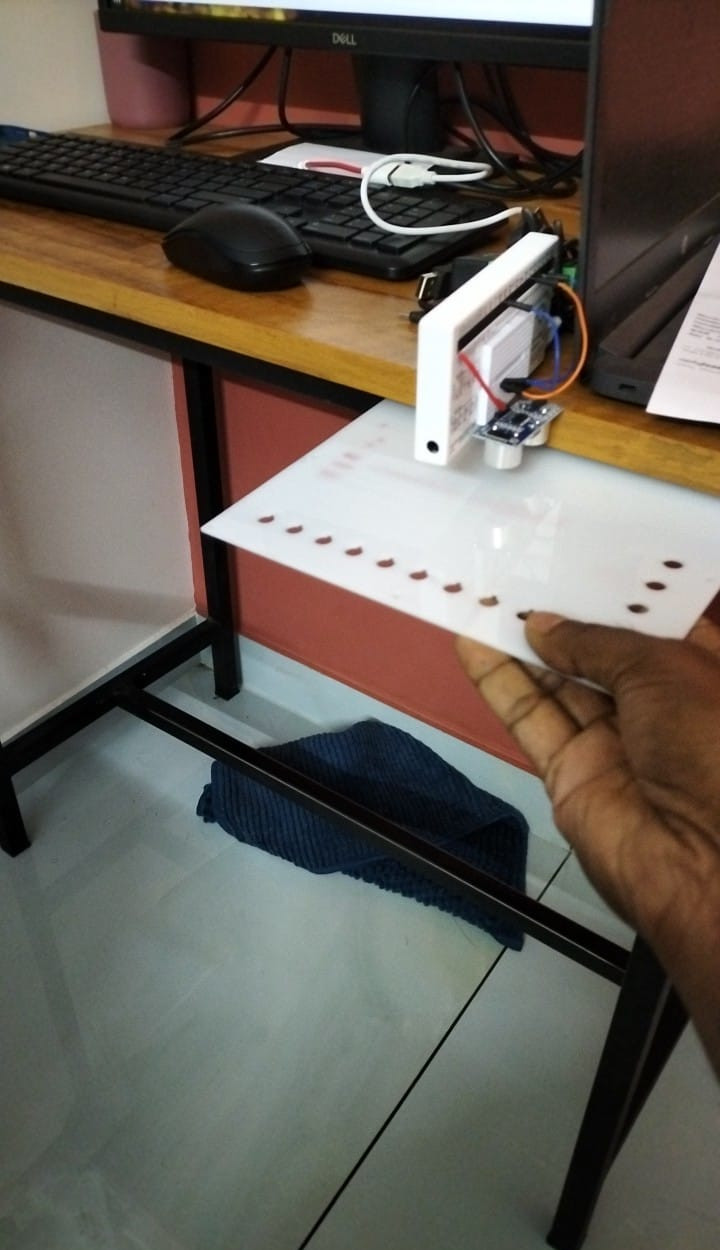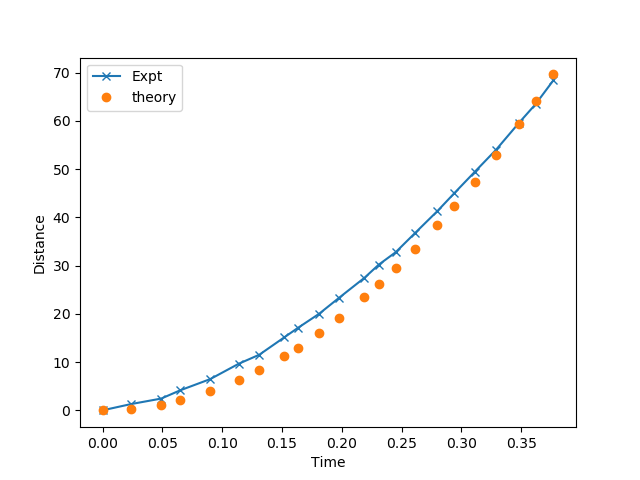# ExpEYES

## Measuring Distance using SR04 Echo Module

Sound pulses gets reflected from hard surfaces. The SR04 distance sensor has two 40 KHz Piezo discs on it. One Transmits and the other receives. The circuitry os powered by 5 volts. Giving a pulse on the trigger input sends a packet of ultrasound pulses and the Echo output is asserted when the reflected pulse is received.

The time interval between the Trigger and Echo are measured. Assuming the speed of sound in air, the distance to the reflecting surface can be calculated.

• Connect Vcc of the SR04 sensor to 5V of SEELab3
• Trig to SQ2
• Echo to IN2
• Ground to any of the Ground terminals

The distance is continuously measured and plotted. From the “Record Data” tab, distance can be measured for a given time interval.### Measurement on a freely falling bodyA flat plate is kept below the SR04 sensor facing downwards, and then released. The distance is measured as a function of time. The measured time is plotted along with the distance calculated using the equation $S = \frac{1}{2}gt^2$.Data collected from the experiment
Data of the region of interest
Python program for plotting the data